Nonparametric Treatment Comparison for Current Status Data | OMICS International
Journal of Biometrics & Biostatistics

Like us on:

# Nonparametric Treatment Comparison for Current Status Data

Yanmin Li1, Adam Shchy2 and Jianguo Sun2*

1Applied Mathematics Institute , Jilin University of Finance and Economics, Changchun, Jilin, 130117, China

2Department of Statistics, University of Missouri, 146 Middlebush Hall, Columbia, Missouri, 65211, USA

*Corresponding Author:
Jianguo Sun
Department of Statistics
University of Missouri
146 Middlebush Hall
Columbia, Missouri, USA
E-mail: [email protected]

Received Date: July 31, 2010; Accepted Date: September 17, 2010; Published Date: September 27, 2010

Citation: Li Y, Shchy A, Sun J (2010) Nonparametric Treatment Comparison for Current Status Data. J Biomet Biostat 1:102. doi:10.4172/2155-6180.1000102

Copyright: © 2010 Li Y, et al. This is an open-access article distributed under the terms of the Creative Commons Attribution License, which permits unrestricted use, distribution, and reproduction in any medium, provided the original author and source are credited.

Visit for more related articles at Journal of Biometrics & Biostatistics

#### Abstract

Current status data occur in many studies and in this case, each subject is observed only once [3,10]. Furthermore, the distributions of observation times may be different for subjects in different treatment groups. This paper focuses on current status recurrent event data that concern occurrence rates of certain recurrent events such as disease infections and discuss nonparmametric comparison of several treatment groups. For the problem, two new tests procedures are proposed and a simulation study is conducted and shows that they are more efficient than the existing ones. An illustrative example on lung tumors is provided.

#### Keywords

Current status data; Nonparametric Treatment Comparison; Recurrent Event Studies; Unequal Observation

#### Introduction

Current status data occur in many studies such as cross-sectional studies, demographic studies, sample surveys and tumorigenicity experiments [3,5,6,9]. In this case, each subject is observed only once and no information is available on subjects between their entry times and observation times. Furthermore, the distributions of observation times may be different for subjects in different treatment groups. In this paper, we will consider such data arising from recurrent event studies that concern occurrence rates of certain recurrent events such as hospitalization and disease infection. For these current status recurrent event data, only the number of the recurrent events of interest that have occurred before the observation time is known and, in particular, the times at which the events occur are unknown.

A typical example of current status data arises from crosssectional studies that are often used in, for example, demographic studies or sample surveys. In these cases, the recurrent event of interest could be giving a birth, getting married, or changing a job. Tumorigenicity experiment is another area that often yields current status data. In these situations, the time until tumor onset is usually of interest and the comparison of different treatments with respect to the rates of development of tumor is often required. The tumor onset time, however, is often not directly observable. Instead, only the death time of animals in the study and the status of tumor onset at or the number of tumors developed by the death time is observed. For the treatment comparison here, an important factor that should be taken into account is animal death time, which serves as observation time and could depend on the treatments. A comparison not accounting for animal death time difference could overestimate or underestimate the treatment difference [6,8,10].

A number of authors have considered the analysis of current status data. For example, Diamond and McDonald  discussed the data arising from demographic studies and Dinse and Lagakos  and Hoel and Walburg  provided some methods for the analysis of the data given by tumorigenicity experiments. Several methods have been proposed for nonparametric treatment comparison based on current status data and these include the procedures given in Andersen and Ronn  and Sun and Kalbfleisch . Also current status data can be regarded as a special case of interval-censored failure time data or panel count data and some nonparametric comparison approaches have been proposed for these situations . However, most of these existing procedures only apply to situations where the distributions of observation times are identical across different treatment groups. One exception that considered the case where the distributions may be different was given by Sun . In the following, two efficient procedures are presented that allow different observation time distributions.

The remainder of the paper is organized as follows. We will first begin with introducing some notation and briefly reviewing the procedures proposed in Sun . Two new procedures are then presented in Section 3 and their asymptotic distributions are given. One procedure, which is much simpler, is designed for the situation in which observation times for all subjects under study follow the same distribution, where the other allows the distributions of observation times to be different or depend on treatments. Section 4 gives some results obtained from a simulation study conducted for assessing the performance of the proposed procedures in practical situations. An illustrative example from a tumorigenicity experiment is also provided in Section 4. Section 5 contains some discussion and concluding remarks.

#### Notation and Existing Procedures

Consider a recurrent event study that consists of n independent subjects and in which each subject receives one of p + 1 different treatments. For subject i, let Ni(t) denote the total number of occurrences of the recurrent event of interest up to time t and define Zi to be the associated p-dimensional vector of the treatment indicators consisting of zero and one, i = 1, ..., n. For example, if there exist four treatments, Zi can be the three-dimensional vector whose j component is one if subject i belongs to treatment group j and zero otherwise, j = 1, 2, 3. Suppose that each subject is observed only once at time Ti. That is, only current status data are available and the observed data are given by {Ni(Ti) , Zi , Ti ; i = 1, ..., n }. In the following, we will assume that the Ti’s are independent of the Ni’s given the Zi’s and the goal is to test the null hypothesis H0 : E [Ni(t) |Zi ] is independent of Zi.

Several procedures are available for testing H0. For example, Sun  suggested to use the following test statistic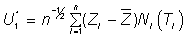assuming that the distributions of the Ti’s are identical for subjects in different treatment groups, where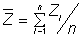. Of course, in practice, the distributions of the observation times Ti’s may depend on the treatment indicators Zi’s. To take this into account, Sun  proposed first to model this dependence by the proportional hazards model λi(t |Zi )= λ0(t)e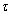1Zi for the hazard function of Ti . Here λ0(t) denotes an unknown baseline hazard function andis a p-dimensional vector of unknown regression parameters.

Note that for the Ti’s, one has the complete failure time data and thus one can easily estimateand the baseline cumulative hazard function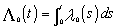by the partial likelihood estimate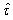Breslow estimate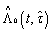respectively. Given these estimates, Sun  proposed to apply the statistic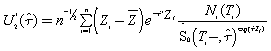for testing H0, where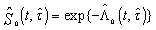. Furthermore, he showed that the statistic asymptotically follows a multivariate normal distribution with mean zero under the null hypothesis H0. In the next section, two more efficient procedures are presented.

Two New Test Procedures

In this section, motivated by the two test procedures discussed in the previous section, we will present two new procedures for testing H0. For this, define µ(t) = E{Ni(t)} under H0 and let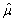(t) denote the isotonic regression estimate of µ(t) [13,11]. To test H0, first suppose that all observation times Ti’s follow the same distribution or the distribution of the Ti’s is independent of the Zi’s. Then by following the statistic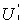, we propose to apply the statistic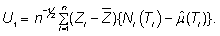It can be easily shown that under H0, the distribution of U1 can be asymptotically approximated by the multivariate normal distribution with mean zero and the covariance matrix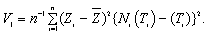Thus one can test H0 by using X1 =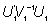whose distribution can be asymptotically approximated by the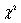distribution with degrees of freedom p.

Now we consider the general situation where the distribution of the Ti’s may depend on the Zi’s. For this, we assume that the dependence can be described by model (1) as in Sun . Letbe defined as before, the partial likelihood estimate ofgiven by the solution to the partial likelihood score equation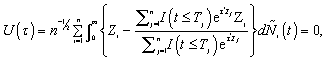where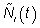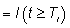To test H0, we propose the following test statistic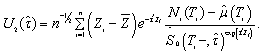It can been seen that the key difference between the existing test statistics reviewed in the previous section and the proposed test statistics is that unlike the former, the latter employs the centered response process Ni(t), thus reducing variance and gaining efficiency. The idea has been used by, for example, Sun  among others.

To describe the asymptotic distribution of U2(), let A()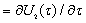and B()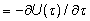Define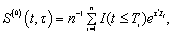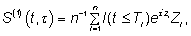and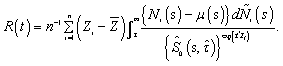Also define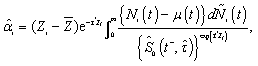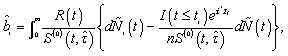and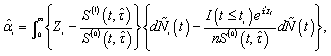i = 1, ..., n. Then one can prove that under H0, the distribution of U2 () can be asymptotically approximated by a multivariate normal distribution with mean 0 and covariance matrix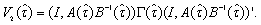Here I denotes the p × p identity matrix and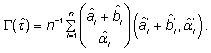The proof follows the similar arguments used in Sun  and is omitted. It follows that the test of hypothesis H0 can be carried out by using the statistic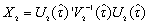whose distribution can be asymptotically approximated by thedistribution with degrees of freedom p.

#### Numerical Results

A simulation study was conducted to assess the performance of the two test procedures presented in the previous section in practical situations. In the study, we considered the two sample comparison problem (p = 1) and took Zi equal to 0 or 1 with probability q. Note that in the design of a study, the sample sizes for two treatment groups are usually set to be equal or close to each other, but in practice, they may be different. We investigated situations with q = 0.50, 0.67 and 0.80. To generate current status data, we first generated the potential number of events from the Poisson distribution with mean 2 and then generated the occurrence times of the events from the uniform distribution. The current status data were thus given by determining how many events have occurred before the observation time generated either from the uniform distribution or exponential distribution with the hazard function given in (1). The results given below are based on 1000 replications.

Table 1 presents the estimated size of the two test procedures proposed in Section 3 with the type I error 0.05 and the total sample size n = 100 or 200. It can be seen that both procedures seem to give the proper size. The estimated powers of the two procedures are given in Table 2. Here we took λ0(t) = e and= 0.5. For the comparison, we also estimated and included in Table 2 the powers of the two test procedures given in Sun  and based on statisticsand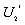, respectively. These two procedures are denoted by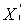and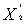and given in brackets in the table. The results indicate that the new procedures always seem to have greater power than the existing procedures and the procedure based on X2 has better power than that based on X1 as expected. Also as expected, the power increases when the sample size increases and the more balance of the sample sizes between the two treatment groups means greater power.

 Sample percentage Procedure X1 Procedure X2 n = 100 n = 200 n = 100 n = 200 q = 50 0.057 0.051 0.047 0.052 q = 67 0.054 0.048 0.052 0.050 q = 80 0.041 0.047 0.054 0.052

Table 1: Estimated size of the proposed test procedures.

 Sample percentage Procedure X1 (X *1 ) Procedure X2 ( X *2) n = 100 n = 200 n = 100 n = 200 q = 50 0.439 (0.325) 0.452 (0.342) 0.703 (0.679) 0.718 (0.701) q = 67 0.413 (0.316) 0.441 (0.338) 0.698 (0.676) 0.711 (0.688) q = 80 0.389 (0.282) 0.426 (0.323) 0.678 (0.661) 0.711 (0.672)

Table 2: Estimated power of the proposed test procedures.

To illustrate the two test procedures given in the previous section, we applied them to the current status data described in Hoel and Walburg  on lung tumors. The data arose from a tumorigenicity experiment on 144 male RFM mice and involve two treatments, conventional environment (96 mice) and germfree environment (48 mice). For each mice, the observation consists of its death time as the observation time and the presence or absence indicator of lung tumor at the death. One of the objectives of the study was to compare the lung tumor incidence rates of the two groups. As shown in Sun , for the data, the death or observation times are quite different between the two treatment groups. That is, we have unequal observation.

For the comparison of the lung tumor incidence rates, define Zi = 0 if the ith animal was in conventional environment and 1 otherwise. The application of the two test procedures described in the previous sections yielded X1 = 8.2549 and X2 = 3.9704 with the corresponding p-values of 0.0041 and 0.0463, respectively, for testing no difference of the lung tumor incidence rates between the two groups. The results suggest that the lung tumor incidence rates between the two treatment groups were significantly different and the animals in the germfree environment had higher incidence than those in the conventional environment. The results above also indicate that in the case where there exist unequal observations, one needs to be careful as the procedure that assumes the equal observation tends to overestimate the treatment difference. These conclusions are similar to those obtained by Sun , which gave the p-values of 0.0009 and 0.028 for the same comparison problem by using the test procedures based on the statisticsand, respectively.

#### Discussion and Concluding Remarks

This paper discussed the nonparametric treatment comparison problem based on current status recurrent event data that usually occur in cross-sectional studies and sample survey that concern occurrence rates of some recurrent events of interest among others. For the problem, a few procedures have been developed under the assumption that the observation time follows the same distribution for all subjects under study [1,6]. However, the assumption may not hold in practice as seen in the example discussed in Section 4. We developed two new nonparametric test procedures that do not require the assumption and have been shown to be more efficient than the existing procedures that do not rely on the assumption.

As mentioned above, current status data discussed here is a special case of panel count data [4,11] and thus the comparison problem discussed here could also occur to panel count data. It is worth noting, however, that the observation processes between the two types of data are quite different. For current status data, the observation process involves only a single time variable, while the observation process with respect to panel count data has to be described by a point process and is thus much more complicated. The focus of this paper has been on recurrent events. If the event can occur only once, current status data become a special case of commonly referred to as interval censored failure time data . As panel count data, interval-censored failure time data involve more than one observation time point for each study subject and thus also have much complex observation processes. For both panel count data and interval-censored failure time data, it would be useful to develop some nonparametric test procedures for treatment comparison that allow different observation processes for subjects in different treatment groups.

A limitation of the proposed test procedures as well as most of existing procedures is that the recurrent event process of interest and the observation process were assumed to be independent given treatments. In some situations, this is not true. An example is given by a tumorigenicity experiment concerning some tumors that are between nonlethal and lethal. In this case, the tumor occurrence rate and the animal death time are correlated and thus their relationship has to be taken into account for the comparison. In general, one usually says that there exists an informative censoring or observation time and some different procedures that take into account the relationship have to be developed for treatment comparison.

#### Acknowledgments

The authors wish to thank two reviewers for their many helpful and thoughtful comments and suggestions that greatly improved the paper.

#### References

Select your language of interest to view the total content in your interested language

### Article Usage

• Total views: 12386
• [From(publication date):
October-2010 - Dec 15, 2019]
• Breakdown by view type
• HTML page views : 8492Can't read the image? click here to refresh